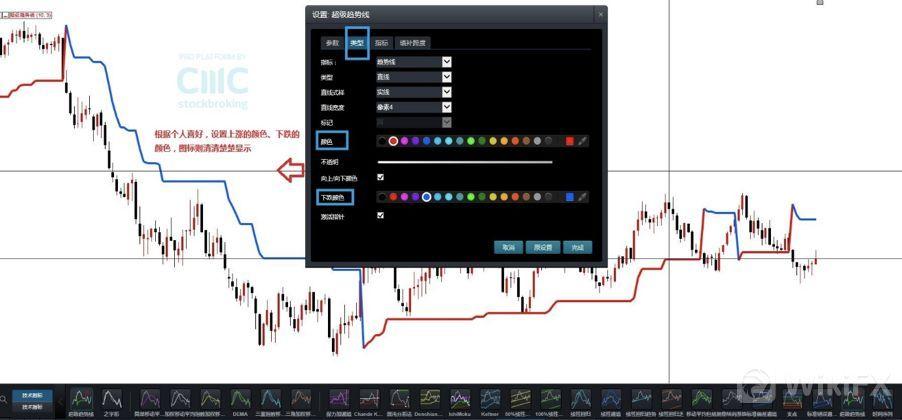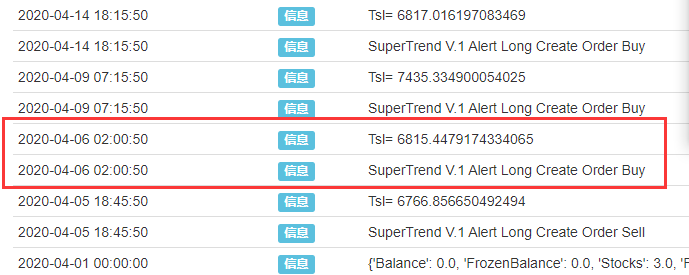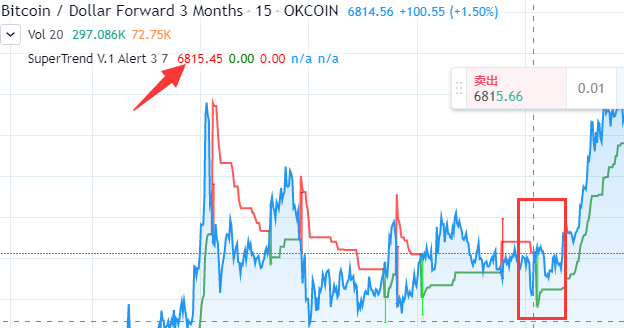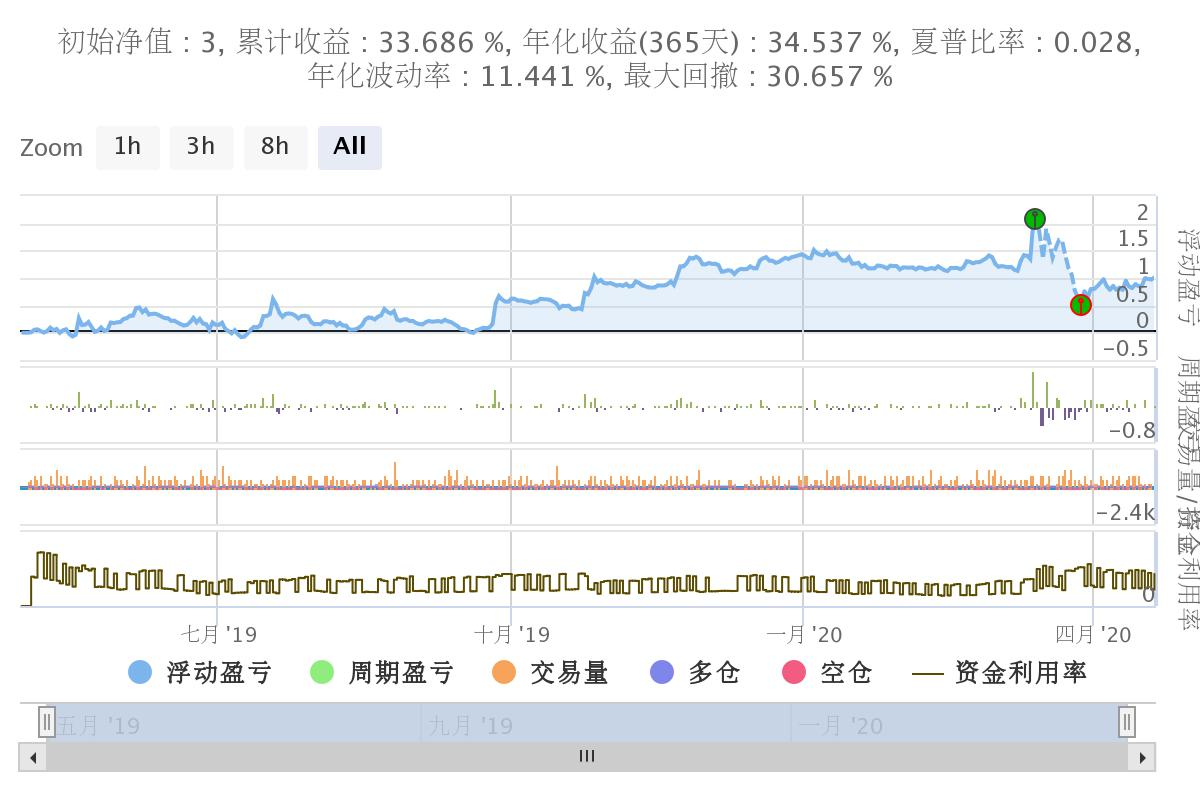# SuperTrend V.1--超级趋势线系统

Author: homily, Created: 2020-04-20 22:10:36, Updated: 2020-06-01 14:07:39

#### 二、系统介绍

CMC Markets 新一代智能交易系统 —— 超级趋势线（Supertrend） 这里有一篇文章介绍这个系统。#### 四、代码转化

1.我们要导入pandas库time库 2.在main函数当中设置使用季度合约（主要跑okex） 3.设定一个循环doTicker()15分钟检测1次。 将代码跑在15分钟的周期上 接着我们在doTicker()中写主要策略。

``````import pandas as pd
import time

def main():
exchange.SetContractType("quarter")
preTime = 0
Log(exchange.GetAccount())
while True:
records = exchange.GetRecords(PERIOD_M15)
if records and records[-2].Time > preTime:
preTime = records[-2].Time
doTicker(records[:-1])
Sleep(1000 *60)

``````

4.我们要取回k线的OHCLV 所以用GetRecords（） 5.我们将取回的数据导入pandas M15 = pd.DataFrame(records) 6.我们要修改表的头部标签。 M15.columns = [‘time’,‘open’,‘high’,‘low’,‘close’,‘volume’,‘OpenInterest’] 其实就是将’open’,‘high’,‘low’,'close’ 的首字母改成小写，便于后期写代码不要一会大写一会小写。

``````def doTicker(records):
M15 = pd.DataFrame(records)
M15.columns = ['time','open','high','low','close','volume','OpenInterest']
``````

7.给数据集合增加一列hl2 hl2=(high+low)/2

``````#HL2
M15['hl2']=(M15['high']+M15['low'])/2

``````

8.接着我们来计算ATR 因为ATR的计算要导入一个变量length，它的取值是Pd

``````M15['prev_close']=M15['close'].shift(1)
``````

``````ranges= [M15['high'] - M15['low'],M15['high']-M15['prev_close'],M15['low']-M15['prev_close']]
``````

``````M15['tr'] = pd.DataFrame(ranges).T.abs().max(axis=1)
``````

``````    alpha = (1.0 / length) if length > 0 else 0.5
M15['atr']=M15['tr'].ewm(alpha=alpha, min_periods=length).mean()
``````

===

``````    #ATR(PD)
length=Pd
M15['prev_close']=M15['close'].shift(1)
ranges= [M15['high'] - M15['low'],M15['high']-M15['prev_close'],M15['low']-M15['prev_close']]
M15['tr'] = pd.DataFrame(ranges).T.abs().max(axis=1)
alpha = (1.0 / length) if length > 0 else 0.5
M15['atr']=M15['tr'].ewm(alpha=alpha, min_periods=length).mean()
``````

9始计算Up和Dn

``````    M15['Up']=M15['hl2']-(Factor*M15['atr'])
M15['Dn']=M15['hl2']+(Factor*M15['atr'])
``````

Up=hl2 -（Factor * atr） Dn=hl2 +（Factor * atr） 是不是很简单呢。

``````TrendUp=close>TrendUp? max(Up,TrendUp) : Up
TrendDown=close<TrendDown? min(Dn,TrendDown) : Dn

Trend = close > TrendDown ? 1: close< TrendUp? -1: nz(Trend,1)
Tsl = Trend==1? TrendUp: TrendDown

linecolor = Trend == 1 ? green : red
``````

``````    M15['TrendUp']=0.0
M15['TrendDown']=0.0
M15['Trend']=1
M15['Tsl']=0.0
M15['linecolor']='Homily'
M15 = M15.fillna(0)
``````

``````    for x in range(len(M15)):
``````

``````        M15['TrendUp'].values[x] = max(M15['Up'].values[x],M15['TrendUp'].values[x-1]) if (M15['close'].values[x-1]>M15['TrendUp'].values[x-1]) else M15['Up'].values[x]
``````

``````        M15['TrendDown'].values[x] = min(M15['Dn'].values[x],M15['TrendDown'].values[x-1]) if (M15['close'].values[x-1]<M15['TrendDown'].values[x-1]) else M15['Dn'].values[x]
``````

``````        M15['Tsl'].values[x] = M15['TrendUp'].values[x] if  (M15['Trend'].values[x]==1) else M15['TrendDown'].values[x]
``````

``````        M15['Tsl'].values[x] = M15['TrendUp'].values[x] if  (M15['Trend'].values[x]==1) else M15['TrendDown'].values[x]
M15['linecolor'].values[x]= 'green' if ( M15['Trend'].values[x]==1) else  'red'
``````

``````    if(M15['Trend'].values[-1] == 1 and M15['Trend'].values[-2] == -1):
if(M15['Trend'].values[-1] == -1 and M15['Trend'].values[-2] == 1):
Log('SuperTrend V.1 Alert Long',"Create Order Sell)

``````

``````    M15['TrendUp']=0.0
M15['TrendDown']=0.0
M15['Trend']=1
M15['Tsl']=0.0
M15['linecolor']='Homily'
M15 = M15.fillna(0)

for x in range(len(M15)):
M15['TrendUp'].values[x] = max(M15['Up'].values[x],M15['TrendUp'].values[x-1]) if (M15['close'].values[x-1]>M15['TrendUp'].values[x-1]) else M15['Up'].values[x]
M15['TrendDown'].values[x] = min(M15['Dn'].values[x],M15['TrendDown'].values[x-1]) if (M15['close'].values[x-1]<M15['TrendDown'].values[x-1]) else M15['Dn'].values[x]
M15['Trend'].values[x] = 1 if (M15['close'].values[x] > M15['TrendDown'].values[x-1]) else ( -1 if (M15['close'].values[x]< M15['TrendUp'].values[x-1])else M15['Trend'].values[x-1] )
M15['Tsl'].values[x] = M15['TrendUp'].values[x] if  (M15['Trend'].values[x]==1) else M15['TrendDown'].values[x]
M15['linecolor'].values[x]= 'green' if ( M15['Trend'].values[x]==1) else  'red'

if(M15['Trend'].values[-1] == 1 and M15['Trend'].values[-2] == -1):
Log('Tsl=',Tsl)
if(M15['Trend'].values[-1] == -1 and M15['Trend'].values[-2] == 1):
Log('SuperTrend V.1 Alert Long',"Create Order Sell)
Log('Tsl=',Tsl)
``````

##### 五、全部代码

``````'''backtest
start: 2019-05-01 00:00:00
end: 2020-04-21 00:00:00
period: 15m
exchanges: [{"eid":"Futures_OKCoin","currency":"BTC_USD"}]
'''

import pandas as pd
import time

def main():
exchange.SetContractType("quarter")
preTime = 0
Log(exchange.GetAccount())
while True:
records = exchange.GetRecords(PERIOD_M15)
if records and records[-2].Time > preTime:
preTime = records[-2].Time
doTicker(records[:-1])
Sleep(1000 *60)

def doTicker(records):
#Log('onTick',exchange.GetTicker())
M15 = pd.DataFrame(records)

#Factor=3
#Pd=7

M15.columns = ['time','open','high','low','close','volume','OpenInterest']

#HL2
M15['hl2']=(M15['high']+M15['low'])/2

#ATR(PD)
length=Pd
M15['prev_close']=M15['close'].shift(1)
ranges= [M15['high'] - M15['low'],M15['high']-M15['prev_close'],M15['low']-M15['prev_close']]
M15['tr'] = pd.DataFrame(ranges).T.abs().max(axis=1)
alpha = (1.0 / length) if length > 0 else 0.5
M15['atr']=M15['tr'].ewm(alpha=alpha, min_periods=length).mean()

M15['Up']=M15['hl2']-(Factor*M15['atr'])
M15['Dn']=M15['hl2']+(Factor*M15['atr'])

M15['TrendUp']=0.0
M15['TrendDown']=0.0
M15['Trend']=1
M15['Tsl']=0.0
M15['linecolor']='Homily'
M15 = M15.fillna(0)

for x in range(len(M15)):
M15['TrendUp'].values[x] = max(M15['Up'].values[x],M15['TrendUp'].values[x-1]) if (M15['close'].values[x-1]>M15['TrendUp'].values[x-1]) else M15['Up'].values[x]
M15['TrendDown'].values[x] = min(M15['Dn'].values[x],M15['TrendDown'].values[x-1]) if (M15['close'].values[x-1]<M15['TrendDown'].values[x-1]) else M15['Dn'].values[x]
M15['Trend'].values[x] = 1 if (M15['close'].values[x] > M15['TrendDown'].values[x-1]) else ( -1 if (M15['close'].values[x]< M15['TrendUp'].values[x-1])else M15['Trend'].values[x-1] )
M15['Tsl'].values[x] = M15['TrendUp'].values[x] if  (M15['Trend'].values[x]==1) else M15['TrendDown'].values[x]
M15['linecolor'].values[x]= 'Long' if ( M15['Trend'].values[x]==1) else  'Short'

linecolor=M15['linecolor'].values[-2]
close=M15['close'].values[-2]
Tsl=M15['Tsl'].values[-2]

if(M15['Trend'].values[-1] == 1 and M15['Trend'].values[-2] == -1):

Log('Tsl=',Tsl)
position = exchange.GetPosition()
if len(position) > 0:
Amount=position["Amount"]
exchange.SetDirection("closesell")

if(M15['Trend'].values[-1] == -1 and M15['Trend'].values[-2] == 1):
Log('SuperTrend V.1 Alert Long','Create Order Sell')
Log('Tsl=',Tsl)
position = exchange.GetPosition()
if len(position) > 0:
Amount=position["Amount"]
exchange.SetDirection("sell")
``````

#### 六、回测与总结#### 六、写在最后

SuperTrend是一个非常不错的交易系统

SuperTrend系统的主要原理是采用ATR通道突破策略（类似于肯特通道） 但其变化的地方主要在于使用了强盗布林的收窄策略，或者说是逆向的唐奇安原理。 在行情运行中不断收窄上下通道。 以便达到通道突破转向的操作。（一旦通道突破，上下轨恢复初始值）

Related

More

zdg4484 YYDS!

lglydz2010 如果直接使用这个策略在OK交易所交易需要怎么连接交易所，小白一个不会python,看不明白

bamsmen 这里如果312那波行情没吃到的话应该参数还有很大的调整空间，因为supertrend主要就是抓趋势单，312是不应该错过的。另外期待lz的pine翻译器早日问世

xunfeng91 pine的翻译器，期待

tiemuer 没啥文化只能说一声 牛逼！

frank131419 “估摸着某一天写一个pine的翻译器。一切皆可python。”—— 牛，好些人看好这个！

Kmeans 回测引擎的代码是否可以开源呢，我想实现复现一下 然后用svm找出最好的参数

dsaidasi 这个系统好像也曾经是收益率前十的期货策略。长期坚持做下去是能赚钱的。

ovels 期待期待，pine真的看不太懂，教程也很少

homily 意思是缺少pandas包 你的系统可能需要pip install pandas

Ant_Sky 请问这是怎么处理的呢？万分感谢

homily 啊哈哈，谢谢老板

lonelyman 求JS版！

homily 恩啊。学习精髓。

homily 是的，完全正确

homily 赞的，！

homily 感谢梦梦老师哈